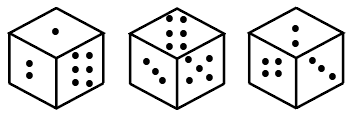Question 3

# Three different positions of the same dice, which has one to six dots on its six faces, are shown below. When one dot is at the bottom the number of dots on the top will be:Solution

From the first position of the dice, 6 is not opposite to 1 and 2

From the second position of the dice, 6 is not opposite to 3 and 5

$$=$$>  6 is opposite to the remaining number 4

From the second position of the dice, 3 is not opposite to 5

From the third position of the dice, 3 is not opposite to 2

$$=$$> 3 is opposite to the remaining number 1

$$\therefore\$$When one dot is at the bottom the number of dots on the top is 3

Hence, the correct answer is Option A